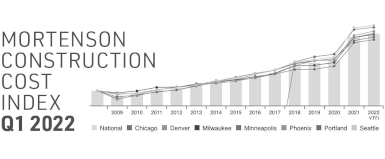# Construction Cost Index: Significance, Calculation, and Trends

Construction projects are complex endeavors influenced by a multitude of factors, including material costs, labor expenses, and market dynamics. To accurately track and assess the changes in construction costs over time, the Construction Cost Index (CCI) plays a crucial role. The CCI is a vital tool for industry professionals, economists, and policymakers, aiding in decision-making, project budgeting, and economic analysis.## Understanding the Construction Cost Index

The Construction Cost Index is a statistical measure used to monitor the variations in the cost of construction over a specified period. It takes into account the price changes of a predefined basket of goods and services that constitute a typical construction project. These components include materials like steel, cement, and lumber, as well as labor costs and various other expenses.

The CCI serves as a valuable indicator of inflation in the construction sector. Just as the Consumer Price Index (CPI) reflects changes in the cost of living for the general population, the CCI focuses specifically on the construction industry. Fluctuations in the index provide insights into how external factors such as supply and demand, economic conditions, and geopolitical events impact construction costs.

## Significance of the Construction Cost Index

The Construction Cost Index holds significant importance for multiple stakeholders:

• Construction Companies: Construction firms utilize the CCI to adjust project budgets and estimates. When prices of construction materials surge, contractors can refer to the index to revise their bids and avoid potential losses.
• Investors: Investors in construction projects use the CCI to assess potential risks and returns. Fluctuations in construction costs can impact the profitability of projects, making the CCI an essential factor in investment decisions.
• Policy Makers: Government entities and policymakers refer to the CCI to analyze economic trends and make informed decisions. The index aids in formulating construction-related policies and regulations.
• Economists: Economists study the CCI to understand broader economic trends. Sharp increases in construction costs might indicate broader inflationary pressures, while decreases could signify economic downturns.

## Calculation of the Construction Cost Index

The Construction Cost Index is calculated using a weighted average formula that considers the relative importance of different cost components. The general formula for calculating the index is:

CCI = Σ ((Pt * Q0) / (P0 * Q0)) * Wt

Where:

• Pt is the price of the item/component in the current period (t).
• P0 is the price of the item/component in the base period (t=0).
• Q0 is the quantity of the item/component in the base period (t=0).
• Wt is the weight of the item/component in the current period (t).

The index is calculated for a specific base period, and any changes in the prices of the components from that base period are reflected in the index over time. The weights assigned to each component reflect their relative importance in the overall construction process.

## Interpreting the Construction Cost Index

The Construction Cost Index is typically expressed as a percentage value relative to the base period. A value above 100% indicates an increase in construction costs compared to the base period, while a value below 100% indicates a decrease.

For example, if the CCI for a given year is 120, it implies that construction costs have increased by 20% compared to the base period. This insight helps stakeholders anticipate cost changes, adjust budgets, and formulate effective strategies.

## Challenges in Construction Cost Index Calculation

While the Construction Cost Index is a valuable tool, its calculation isn't without challenges. One significant challenge lies in selecting an appropriate base period. The base period should represent a stable point in time, and changes in construction costs should be prominent enough to warrant tracking over time.

Additionally, obtaining accurate and up-to-date data for the prices and quantities of various components is crucial. Fluctuations in data quality can lead to misleading index values. Data collection from various sources such as suppliers, contractors, and government agencies can introduce discrepancies.

## Real-World Application of the Construction Cost Index

Let's consider a hypothetical scenario to illustrate the significance of the Construction Cost Index.

ABC Construction Company is planning to undertake a new residential building project. They estimate the materials and labor costs based on current market rates. However, during the construction phase, they notice a significant increase in the cost of steel and cement, two essential components of their project.

By referring to the Construction Cost Index, ABC Construction Company can identify that the index has risen by 15% since the base period. Armed with this information, they can adjust their budget and project estimates accordingly. Without the CCI, they might have faced budget overruns and financial challenges mid-project.